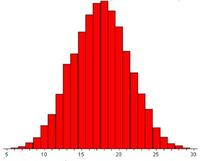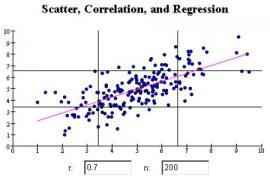MERLOT Biology Portal Visit Main MERLOTMerlot Biology Pedagogic CollectionBelow we provide information and links to topics in statistics that may be appropriate for your students. The Glossary from HyperStat is also a potentially useful resource. Basic statistical calculations, such as the calculation of the mean, variance, and standard deviation, are fundamental to the analysis of most data. Learn more here The central limit theorem is intimately related to the idea that an increase in sample size improves the estimate for the mean value of a population Learn more here Covariance and correlation between two data sets (bivariate data) may also be appropriately introduced in your course. Learn more here The t-distribution and t-statistics are useful in establishing confidence limits (error bars) for a sample mean, or for the slope and intercept values calculated from a least squares linear regression. Learn more here Least Squares Linear Regression is often introduced in introductory courses to varying degrees of mathematical sophistication. Learn more here Fourier transforms of time series or spatial data are used throughout the geosciences. Consider providing your introductory-level students with some familiarity of this essential analysis tool. Learn more here